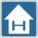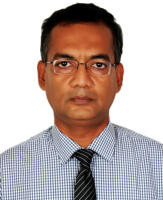Md. Zahurul Haq, Ph.D., CEA, FBSME, FIEB Professor Department of Mechanical Engineering BUET, Dhaka, BangladeshSyllabus                           3.00 Credit Hours Introduction     to     classical     and     statistical     viewpoints     in     thermodynamics;     Concepts     of equilibrium,    stability,    reversibility,    irreversibility    and        availability;    Concepts    of    entropy; Principle   of   increase   of   entropy;   Calculation   of   entropy   changes;   Statistical   interpretation; Entropy   of   mixing;   Absolute   entropy;   Entropy   flow   and   entropy   production;   Properties   of   pure substances;   Ideal   gases;   Ideal   gas   mixtures   of   constant   composition;   Ideal   gas   mixtures   of variable   compositions;   Thermodynamic   potentials:   Helmholtz   free   energy   functions,   Gibbs free     energy     function;     Application     of     free     energy     functions;     Transformations     and thermodynamic    potentials;    Maxwell    relations;    Phase    transitions;    The    Clausius-Clapeyron equation;     Statistical     mechanics:     fundamental     principles,     energy     states     and     levels; Thermodynamic   probability:   Bose-Einstein   statistics,   Fermi-Dirac   statistics;   Thermodynamic properties   of   a   system;   Special   Topics:   elastic   systems,   fuel   cells,   magnetic   systems,   thermo- electricity.
Course Notes [2021-22]: Course Outline A. Classical Thermodynamics 1 cr. hr.     1. EOS & Thermodynamic property relationships                  ,      2. Thermodynamics of homogeneous mixtures                      3. Exergy                                  4. Chemical Equilibrium                                                       B. Statistical thermodynamics                                                2 cr. hr.     Statistical Thermodynamics will be covered by Dr. Kazi Arafat RahmanReference/Text Books: K. Wark (1995), Advanced Thermodynamics for Engineers, McGraw-Hill. T. Engel & P. Reid (2013), Thermodynamics, Statistical Thermodynamics, & Kinetics, Pearson. M.W. Zemansky & R.H. Dittman (1981), Heat and Thermodynamics, McGraw-Hill. F.W. Sears & G. L. Salinger (1975), Thermodynamics, Kinetic Theory, and Statistical Thermodynamics, Addison-Wesley.
Notices: Assignments: ,